Documentation

### This is machine translation

Mouseover text to see original. Click the button below to return to the English version of the page.

## Using Simulink Function Blocks and Exported Stateflow Functions

Simulink® functions have an interface with input and output arguments similar to programming languages. You create the function definitions:

• Graphically, using Simulink blocks in a Simulink Function block, or Stateflow® state transitions in a graphical function exported from a Stateflow chart.

• Textually, using MATLAB® code in a MATLAB function exported from a Stateflow chart.

Set up a Simulink Function block to receive data through an input argument from a function caller. Multiply the input argument by 2, and then pass the calculated value back through an output argument.

Open the Library Browser by selecting the Library Browser toolbar button.

2. On the block, enter this function prototype to set the function name to `timestwo`, the input argument to `u`, and the output argument to `y`:

`y = timestwo(u)`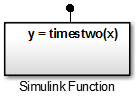3. Double-click the block to open the subsystem defining the function.

4. Add a Gain block and set the Gain parameter to `2`.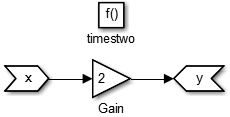#### Export Graphical Function from Stateflow Chart

Set up a graphical function in a Stateflow chart to receive data through an input argument from a function caller. Multiply the input argument by 2, and then pass the calculated value back through an output argument. Set chart parameters to export the function to a Simulink model.

Define a Graphical Function.  Create a graphical function in a Stateflow chart. Define the function interface and function definition.

2. Drag the new chart to your model. Double-click to open the chart.

3. Add a graphical function. From the left-side toolbar, click and drag the graphical function icon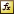onto the chart.

4. Define the function interface. In the function box, replace the `?` with the function interface ```y = timestwo_gf(x)```.

5. Define the function. Click the transition arrow and replace the `?` with the MATLAB code `{y = 2*x}`.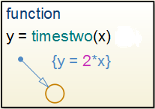6. Add a transition in the chart. Connect the chart input to a Sine Wave input and the output to a Scope.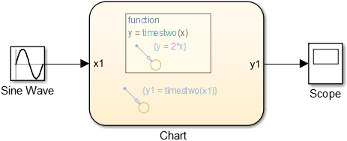7. Run a simulation to test the function.

Set Argument Parameters for a Graphical Function.  Specify the size, complexity, and type of the function input and output arguments. A chart can export only functions with fully specified prototypes.

1. Open Model Explorer. In the left pane, select the graphical function.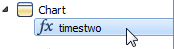2. From the Column View list in the middle pane, select `Stateflow`. Select the filter icon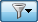, and then select All Stateflow Objects. From the table at the bottom of the pane, select an input or output argument.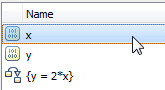3. In the right pane, enter `1` (scaler) for Size, select `Off` (real number) for Complexity, and select `double` for Type.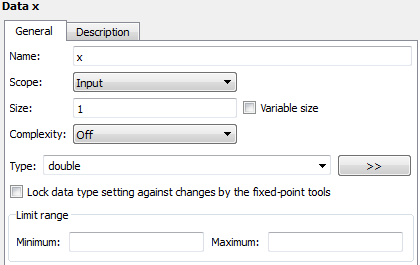4. Repeat steps `2` and `3` with the remaining function arguments.

Set Export Function Parameters for a Graphical Function.  Set parameters to export the graphical function during a simulation.

1. From the left pane, select the chart containing the graphical function.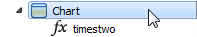Alternatively, open the chart and from the menu, select Chart > Properties.

2. In the property dialog box, select Export Chart Level Functions (Make Global) and Allow exported functions to be called by Simulink check boxes.

If you are calling the exported graphical function from another Stateflow chart (not the chart that exported the graphical function), you do not need to select the Allow exported functions to be called by Simulink check box.

#### Export MATLAB Function from Stateflow Chart

Set up a MATLAB function in a Stateflow chart to receive data through an input argument from a function caller. Multiply the input argument by 2, and then pass the calculated value back through an output argument. Set chart parameters to export the function to a Simulink model.

Define MATLAB Function.  Create a graphical function in a Stateflow chart. Define the function interface and function definition.

1. Add a Stateflow Chart to your Simulink model. From the Simulink Editor menu, select File > New > Chart. A new chart opens in a new model.

2. Drag the new chart to your model. Double-click the chart to open it.

3. Add a graphical function. From the left-side toolbar, click and drag the graphical function icononto the chart.

4. Define the function interface. In the function box, replace the `?` with the function interface ```y = timestwo_ml(x)```.5. Double-click the function box to open the MATLAB code editor. Define the function with the MATLAB code.6. Add a transition to test the MATLAB function. Add a Sine Wave input and connect the output to a Scope.7. Run a simulation to test the function.

Set Argument Parameters.  Specify the size, complexity, and type of the function input and output arguments. A chart can export only functions with fully specified prototypes.

1. Open Model Explorer. In the left pane, select the MATLAB function.2. In the middle pain, from the Column View list, select `Stateflow`. From the table, select an input or output argument.3. In the right pane, enter `1` (scaler) for Size, select `Off` (real number) for Complexity, and select `double` for Type.4. Repeat steps `2` and `3` with the remaining function arguments.

Set Export Function Parameters.  Set parameters to export the MATLAB function during a simulation.

1. From the left pane, select the chart containing the graphical function.Alternatively, open the chart and from the menu, select Chart > Properties.

2. In the property dialog box, select Export Chart Level Function (Make Global) and Allow exported functions to be called by Simulink check boxes.

If you are calling the exported graphical function from another Stateflow chart (not the chart that exported the graphical function), you do not need to select the Allow exported functions to be called by Simulink check box.

### Call Simulink Functions with a Function Caller Block

The following examples show how to use a Function Caller block to call:

• Exported Graphical function from a Stateflow chart.

• Exported MATLAB function from a Stateflow chart.

The functions multiply a value from the caller by `2`, and then send the calculated value back to the caller.

Because the Function Caller block cannot determine function arguments at compile time, you have to name the Function Caller block input and output arguments. The argument names in the Function prototype must match the function argument names.

#### Function Caller Block to Call Simulink Function Block

Set up a Function Caller block to send data through an input argument to a Simulink Function block. Receive data back from the function through an output argument. Points to consider:

To add a Function Caller block.

1. Add a Function Caller block from the User-Defined Functions library into your model.

2. In the Function Caller dialog box, in the Function prototype box, enter `y = timestwo(u)`. This function prototype creates an input port `u` and output port `y` on the Function Caller block.

### Note

Typing in a blank text box displays a list of previously created function prototypes that match the text you are typing.

3. Add a Sine Wave block to the input and a Scope block to the output.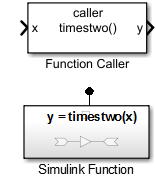4. Set up a Simulink Function block as described in Simulink Function Block.

Simulate the Model.  After you create a function using a Simulink Function block and setup a call to that function using a Function Caller block, you can simulate the model.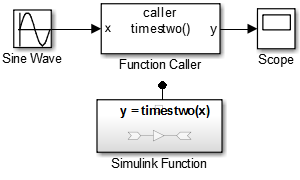2. Run a simulation.

3. To view the signal results, double-click the Scope block. The input sine wave with an amplitude of `2` is doubled.#### Function Caller Block to Call Exported Graphical Function

Calling an exported graphical function from Stateflow is no different than calling a Simulink Function block. Points to consider:

#### Function Caller Block to Call Exported MATLAB Functions

Calling an exported MATLAB function from Stateflow is no different than calling a Simulink Function block. Points to consider:

### Call Simulink Functions from a MATLAB Function Block

A MATLAB Function block can call another function. The following examples show how to use a MATLAB Function block to call:

• Exported Graphical function from a Stateflow chart.

• Exported MATLAB function from a Stateflow chart.

The functions multiply a value from the caller by `2`, and then send the calculated value back to the caller.

MATLAB Function blocks only support discrete and fixed-in-minor sample times. When using a Sine Wave block as an input signal, setup the model and block parameters for a sample time compatible with the MATLAB Function block:

• Discrete — Open the model Configuration Parameters dialog box to the Solver pane. Set Type to Fixed-step and enter a value for Fixed-step size (fundamental sample time). Open the Sine Wave Block Parameters dialog box and set Sample time to the model sample time you entered.

• Fixed-In-Minor step — Open the Sine Wave Block Parameters dialog box. In the Sample time box, enter `[0 1]`.

#### MATLAB Function Block to Call Simulink Function Block

Set up a MATLAB Function block to send data through an input argument to a Simulink Function block. Receive data back from the function through an output argument.

2. Double-click the block, which opens the MATLAB editor. Enter the function call ```y1 = timestwo(u1)```.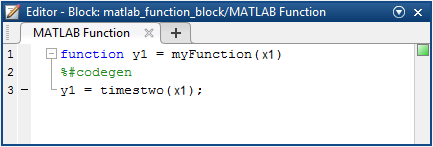### Note

The argument names for the function you define in the MATLAB Function block do not have to match the argument names for the function that you define in the Simulink Function block. For a Function Caller block that calls a Simulink Function block, argument names must match.

3. Add a Sine Wave block for an input signal and a Scope block to view the output signal.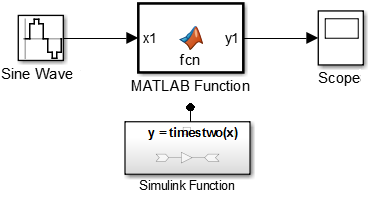4. For the Sine Wave block, set the Sample time to `0.01`. For the model, open the Configuration Parameters dialog box to the solver pane. Set Type to `Fixed-step` and Fixed-step size to `0.01`.

5. Set up a Simulink Function block as described in Simulink Function Block.

#### MATLAB Function Block to Call Exported Graphical Function

Calling an exported graphical function from a MATLAB Function block is the same as calling a Simulink Function block.#### MATLAB Function Block to Call Exported MATLAB Function

Calling an exported MATLAB function from a MATLAB Function block is the same as calling a Simulink Function block.### Call Simulink Functions from a Stateflow Chart

The following examples show how to use a Stateflow chart to call functions external to the chart but accessible in a Simulink model:

• Exported Stateflow graphical function

• Exported Stateflow MATLAB function

There are no limitations to a function call from a Stateflow chart:

• Caller argument names can be different from the function argument names.

• Input signals to a Stateflow chart can be either continuous or discrete.

#### Create Stateflow Chart with a Transition

1. Add a Stateflow Chart to your Simulink model. From the Simulink Editor menu, select File > New > Chart. A new chart opens in a new model.

2. Drag the new chart to your model. Double-click the chart to open it.

3. Add a graphical function. From the left-side toolbar, click and drag the transition icon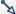onto the chart.

2. In the Data dialog box, set Name to `x1`.

2. In the Data dialog box, set Name to `y1`.

6. Add a Sine Wave block and connect signal output to the chart input port. Add a Scope block and connect input to the chart output port.

7. Edit transition code to call a function. For example, to call the Simulink Function block, enter:

`{y1=timestwo_sf(x1);}`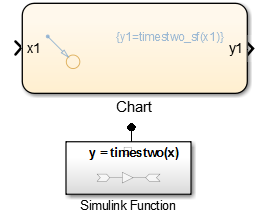8. Set up a Simulink Function block as described in Simulink Function Block.

Stateflow Chart to Call Exported Graphical Function.  Calling an exported graphical function from a Stateflow chart is the same as calling a Simulink Function block.

1. Open a Stateflow chart.

2. Click transition and enter

`{y2=timestwo_gf(x2);}`Stateflow Chart to Call Exported MATLAB Functions.  Calling an exported MATLAB function from a Stateflow chart is the same as calling a Simulink Function block.

1. Open Stateflow chart.

2. Click transition and enter:

`{y3=timestwo_sf(x3);}`### Call a Simulink Function Block from Multiple Sites

If you call a Simulink Function block from multiple sites, all call sites share the state of the function. For example, suppose that you have a Stateflow chart with two calls and two Function Caller blocks with calls to the same function.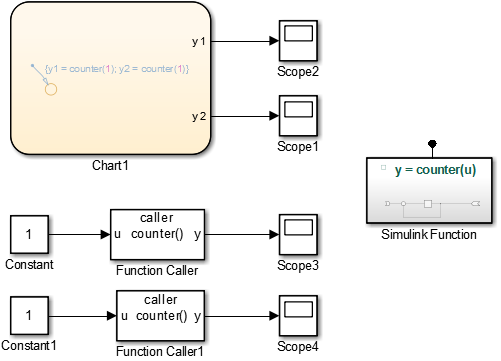A function defined with a Simulink Function block is a counter that increments by `1` each time it is called with an input of `1`.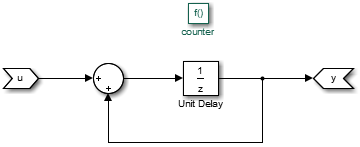The Unit Delay block has state because the block value is persistent between calls from the two Function Caller blocks and the Stateflow chart. Conceptually, you can think of this function being implemented in MATLAB code:

```function y = counter(u) persistent state; if isempty(state) state = 0; end y = state; state = state + u;```

Simulink initializes the state value of the Unit Delay block at the beginning of a simulation. After that, each time the function is called, the state value is updated.

In this example, the output observed in Scope1 increments by `4` at each time step. Scope2, Scope3, and Scope4 show a similar behavior. The only difference is a shift in the observed signal due to the execution sequence of the function calls.#### Diagnostic Settings With Multiple Callers

For multiple callers that share a function and have different sample time rates, data integrity and consistency of real-time code might be a problem. Consider controlling the severity of diagnostics.

Select a Fixed-step solver. Set the Treat each discrete rate as a separate task to:

• Clear (single–tasking), and then set Single task rate transition parameter to `none` (default), `warning`, or `error`.

• Select (multi-tasking), and then set Multitask rate transition parameter to `error` (default) or `warning`.